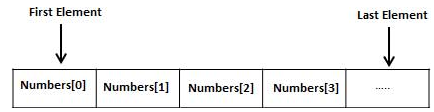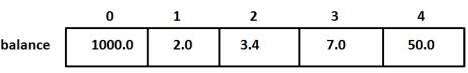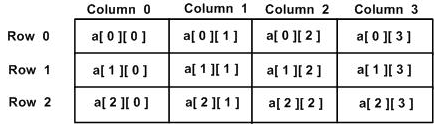Courses

# C: Arrays Computer Science Engineering (CSE) Notes | EduRev

## Computer Science Engineering (CSE) : C: Arrays Computer Science Engineering (CSE) Notes | EduRev

The document C: Arrays Computer Science Engineering (CSE) Notes | EduRev is a part of the Computer Science Engineering (CSE) Course GATE Computer Science Engineering(CSE) 2022 Mock Test Series.
All you need of Computer Science Engineering (CSE) at this link: Computer Science Engineering (CSE)

Arrays a kind of data structure that can store a fixed-size sequential collection of elements of the same type. An array is used to store a collection of data, but it is often more useful to think of an array as a collection of variables of the same type.

Instead of declaring individual variables, such as number0, number1, ..., and number99, you declare one array variable such as numbers and use numbers, numbers, and ..., numbers to represent individual variables. A specific element in an array is accessed by an index.

All arrays consist of contiguous memory locations. The lowest address corresponds to the first element and the highest address to the last element.## Declaring Arrays

To declare an array in C, a programmer specifies the type of the elements and the number of elements required by an array as follows −

`type arrayName [ arraySize ];`

This is called a single-dimensional array. The arraySize must be an integer constant greater than zero and type can be any valid C data type. For example, to declare a 10-element array called balance of type double, use this statement −

`double balance;`

The number of values between braces { } cannot be larger than the number of elements that we declare for the array between square brackets [ ].

If you omit the size of the array, an array just big enough to hold the initialization is created. Therefore, if you write −

`double balance[] = {1000.0, 2.0, 3.4, 7.0, 50.0};`

You will create exactly the same array as you did in the previous example. Following is an example to assign a single element of the array −

`balance = 50.0;`

The above statement assigns the 5th element in the array with a value of 50.0. All arrays have 0 as the index of their first element which is also called the base index and the last index of an array will be total size of the array minus 1. Shown below is the pictorial representation of the array we discussed above −'## Accessing Array Elements

An element is accessed by indexing the array name. This is done by placing the index of the element within square brackets after the name of the array. For example −

`double salary = balance;`

The above statement will take the 10th element from the array and assign the value to salary variable. The following example Shows how to use all the three above mentioned concepts viz. declaration, assignment, and accessing arrays −

`#include <stdio.h> int main () {   int n[ 10 ]; /* n is an array of 10 integers */   int i,j;    /* initialize elements of array n to 0 */            for ( i = 0; i < 10; i++ ) {      n[ i ] = i + 100; /* set element at location i to i + 100 */   }      /* output each array element's value */   for (j = 0; j < 10; j++ ) {      printf("Element[%d] = %d", j, n[j] );   }    return 0;}`

When the above code is compiled and executed, it produces the following result −

`Element = 100Element = 101Element = 102Element = 103Element = 104Element = 105Element = 106Element = 107Element = 108Element = 109`

## Arrays in Detail

Arrays are important to C and should need a lot more attention. The following important concepts related to array should be clear to a C programmer −

 S.N. Concept & Description 1 Multi-dimensional arraysC supports multidimensional arrays. The simplest form of the multidimensional array is the two-dimensional array. 2 Passing arrays to functionsYou can pass to the function a pointer to an array by specifying the array's name without an index. 3 Return array from a function C allows a function to return an array. 4 Pointer to an array You can generate a pointer to the first element of an array by simply specifying the array name, without any index.

## Multi-dimensional Arrays

C programming language allows multidimensional arrays. Here is the general form of a multidimensional array declaration −

`type name[size1][size2]...[sizeN];`

For example, the following declaration creates a three dimensional integer array −

`int threedim;`

## Two-dimensional Arrays

The simplest form of multidimensional array is the two-dimensional array. A two-dimensional array is, in essence, a list of one-dimensional arrays. To declare a two-dimensional integer array of size [x][y], you would write something as follows −

`type arrayName [ x ][ y ];`

Where type can be any valid C data type and arrayName will be a valid C identifier. A two-dimensional array can be considered as a table which will have x number of rows and y number of columns. A two-dimensional array a, which contains three rows and four columns can be shown as follows −Thus, every element in the array a is identified by an element name of the form a[ i ][ j ], where 'a' is the name of the array, and 'i' and 'j' are the subscripts that uniquely identify each element in 'a'.

## Initializing Two-Dimensional Arrays

Multidimensional arrays may be initialized by specifying bracketed values for each row. Following is an array with 3 rows and each row has 4 columns.

`int a = {     {0, 1, 2, 3} ,   /*  initializers for row indexed by 0 */   {4, 5, 6, 7} ,   /*  initializers for row indexed by 1 */   {8, 9, 10, 11}   /*  initializers for row indexed by 2 */};`

The nested braces, which indicate the intended row, are optional. The following initialization is equivalent to the previous example −

`int a = {0,1,2,3,4,5,6,7,8,9,10,11};`

## Accessing Two-Dimensional Array Elements

An element in a two-dimensional array is accessed by using the subscripts, i.e., row index and column index of the array. For example −

`int val = a;`

The above statement will take the 4th element from the 3rd row of the array. You can verify it in the above figure. Let us check the following program where we have used a nested loop to handle a two-dimensional array −

`#include <stdio.h> int main () {   /* an array with 5 rows and 2 columns*/   int a = { {0,0}, {1,2}, {2,4}, {3,6},{4,8}};   int i, j;    /* output each array element's value */   for ( i = 0; i < 5; i++ ) {      for ( j = 0; j < 2; j++ ) {         printf("a[%d][%d] = %d", i,j, a[i][j] );      }   }      return 0;}`

When the above code is compiled and executed, it produces the following result −

`a: 0a: 0a: 1a: 2a: 2a: 4a: 3a: 6a: 4a: 8`

As explained above, you can have arrays with any number of dimensions, although it is likely that most of the arrays you create will be of one or two dimensions.

## Passing Arrays as Function Arguments

If you want to pass a single-dimension array as an argument in a function, you would have to declare a formal parameter in one of following three ways and all three declaration methods produce similar results because each tells the compiler that an integer pointer is going to be received. Similarly, you can pass multi-dimensional arrays as formal parameters.

## Way-1

Formal parameters as a pointer −

`void myFunction(int *param) {   .   .   .}`

## Way-2

Formal parameters as a sized array −

`void myFunction(int param) {   .   .   .}`

## Way-3

Formal parameters as an unsized array −

`void myFunction(int param[]) {   .   .   .}`

## Example

Now, consider the following function, which takes an array as an argument along with another argument and based on the passed arguments, it returns the average of the numbers passed through the array as follows −

`double getAverage(int arr[], int size) {   int i;   double avg;   double sum = 0;   for (i = 0; i < size; ++i) {      sum += arr[i];   }   avg = sum / size;   return avg;}`

Now, let us call the above function as follows −

`#include <stdio.h> /* function declaration */double getAverage(int arr[], int size);int main () {   /* an int array with 5 elements */   int balance = {1000, 2, 3, 17, 50};   double avg;   /* pass pointer to the array as an argument */   avg = getAverage( balance, 5 ) ;    /* output the returned value */   printf( "Average value is: %f ", avg );       return 0;}`

When the above code is compiled together and executed, it produces the following result −

`Average value is: 214.400000`

As you can see, the length of the array doesn't matter as far as the function is concerned because C performs no bounds checking for formal parameters.

## Return array from function

C programming does not allow to return an entire array as an argument to a function. However, you can return a pointer to an array by specifying the array's name without an index.

If you want to return a single-dimension array from a function, you would have to declare a function returning a pointer as in the following example −

`int * myFunction() {   .   .   .}`

Second point to remember is that C does not advocate to return the address of a local variable to outside of the function, so you would have to define the local variable as static variable.

Now, consider the following function which will generate 10 random numbers and return them using an array and call this function as follows −

`#include <stdio.h>/* function to generate and return random numbers */int * getRandom( ) {   static int  r;   int i;   /* set the seed */   srand( (unsigned)time( NULL ) );     for ( i = 0; i < 10; ++i) {      r[i] = rand();      printf( "r[%d] = %d", i, r[i]);   }   return r;}/* main function to call above defined function */int main () {   /* a pointer to an int */   int *p;   int i;   p = getRandom();	   for ( i = 0; i < 10; i++ ) {      printf( "*(p + %d) : %d", i, *(p + i));   }   return 0;}`

When the above code is compiled together and executed, it produces the following result −

`r = 313959809r = 1759055877r = 1113101911r = 2133832223r = 2073354073r = 167288147r = 1827471542r = 834791014r = 1901409888r = 1990469526*(p + 0) : 313959809*(p + 1) : 1759055877*(p + 2) : 1113101911*(p + 3) : 2133832223*(p + 4) : 2073354073*(p + 5) : 167288147*(p + 6) : 1827471542*(p + 7) : 834791014*(p + 8) : 1901409888*(p + 9) : 1990469526`

## Pointer to an Array

It is most likely that you would not understand this section until you are through with the chapter 'Pointers'.

Assuming you have some understanding of pointers in C, let us start: An array name is a constant pointer to the first element of the array. Therefore, in the declaration −

`double balance;`

balance is a pointer to &balance, which is the address of the first element of the array balance. Thus, the following program fragment assigns p as the address of the first element of balance

`double *p;double balance;p = balance;`

It is legal to use array names as constant pointers, and vice versa. Therefore, *(balance + 4) is a legitimate way of accessing the data at balance.

Once you store the address of the first element in 'p', you can access the array elements using *p, *(p+1), *(p+2) and so on. Given below is the example to show all the concepts discussed above −

`#include <stdio.h>int main () {   /* an array with 5 elements */   double balance = {1000.0, 2.0, 3.4, 17.0, 50.0};   double *p;   int i;   p = balance;    /* output each array element's value */   printf( "Array values using pointer");	   for ( i = 0; i < 5; i++ ) {      printf("*(p + %d) : %f",  i, *(p + i) );   }   printf( "Array values using balance as address");	   for ( i = 0; i < 5; i++ ) {      printf("*(balance + %d) : %f",  i, *(balance + i) );   }    return 0;}`

When the above code is compiled and executed, it produces the following result −

`Array values using pointer*(p + 0) : 1000.000000*(p + 1) : 2.000000*(p + 2) : 3.400000*(p + 3) : 17.000000*(p + 4) : 50.000000Array values using balance as address*(balance + 0) : 1000.000000*(balance + 1) : 2.000000*(balance + 2) : 3.400000*(balance + 3) : 17.000000*(balance + 4) : 50.000000`

In the above example, p is a pointer to double, which means it can store the address of a variable of double type. Once we have the address in p, *p will give us the value available at the address stored in p, as we have shown in the above example.

Offer running on EduRev: Apply code STAYHOME200 to get INR 200 off on our premium plan EduRev Infinity!

## GATE Computer Science Engineering(CSE) 2022 Mock Test Series

131 docs|159 tests

,

,

,

,

,

,

,

,

,

,

,

,

,

,

,

,

,

,

,

,

,

;